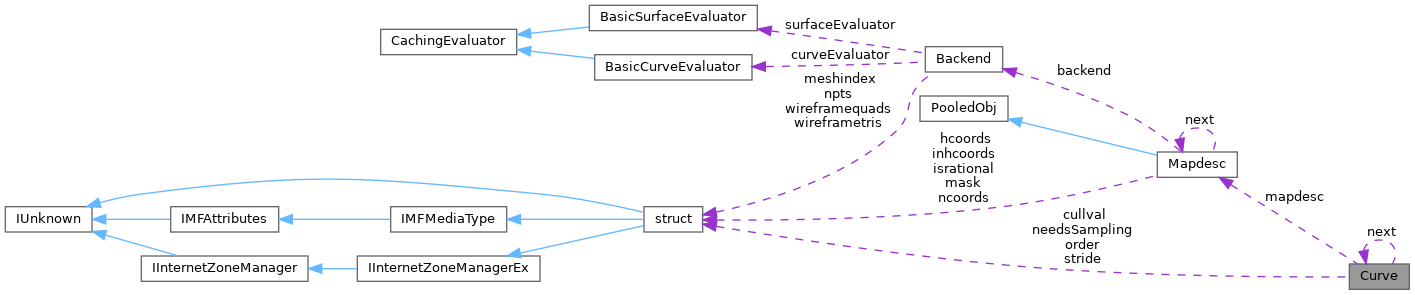ReactOS 0.4.15-dev-7076-gc00d41d
Curve Class Reference

`#include <curve.h>`

Collaboration diagram for Curve:[legend]

## Public Member Functions

Curve (Quilt *, REAL, REAL, Curve *)

Curve (Curve &, REAL, Curve *)

Curvenext

## Private Member Functions

void clamp (void)

void setstepsize (REAL)

void getstepsize (void)

int cullCheck (void)

int needsSamplingSubdivision (void)

## Private Attributes

Mapdescmapdesc

int stride

int order

int cullval

int needsSampling

REAL cpts [MAXORDER *MAXCOORDS]

REAL spts [MAXORDER *MAXCOORDS]

REAL stepsize

REAL minstepsize

REAL range 

class Curvelist

## Detailed Description

Definition at line 46 of file curve.h.

## ◆ Curve() [1/2]

 Curve::Curve ( Quilt * , REAL , REAL , Curve * )

## ◆ Curve() [2/2]

 Curve::Curve ( Curve & upper, REAL value, Curve * c )

Definition at line 101 of file curve.cc.

102{
103 Curve &lower = *this;
104
105 lower.next = c;
106 lower.mapdesc = upper.mapdesc;
107 lower.needsSampling = upper.needsSampling;
108 lower.order = upper.order;
109 lower.stride = upper.stride;
110 lower.cullval = upper.cullval;
111
112 REAL d = (value - upper.range) / upper.range;
113
114 if( needsSampling )
115 mapdesc->subdivide( upper.spts, lower.spts, d, upper.stride, upper.order );
116
117 if( cullval == CULL_ACCEPT )
118 mapdesc->subdivide( upper.cpts, lower.cpts, d, upper.stride, upper.order );
119
120 lower.range = upper.range;
121 lower.range = value;
122 lower.range = value - upper.range;
123 upper.range = value;
124 upper.range = upper.range - value;
125}
Definition: curve.h:46
REAL cpts[MAXORDER *MAXCOORDS]
Definition: curve.h:58
REAL range
Definition: curve.h:62
REAL spts[MAXORDER *MAXCOORDS]
Definition: curve.h:59
int cullval
Definition: curve.h:56
int order
Definition: curve.h:55
Mapdesc * mapdesc
Definition: curve.h:53
int needsSampling
Definition: curve.h:57
int stride
Definition: curve.h:54
Curve * next
Definition: curve.h:51
void subdivide(REAL *, REAL *, REAL, int, int)
Definition: mapdesc.cc:577
#define CULL_ACCEPT
Definition: defines.h:42
float REAL
Definition: types.h:41
#define d
Definition: ke_i.h:81
#define c
Definition: ke_i.h:80
Definition: pdh_main.c:94

## ◆ clamp()

 void Curve::clamp ( void )
private

Definition at line 134 of file curve.cc.

135{
136 if( stepsize < minstepsize )
138}
REAL stepsize
Definition: curve.h:60
REAL minstepsize
Definition: curve.h:61
REAL clampfactor
Definition: mapdesc.h:107

## ◆ cullCheck()

 int Curve::cullCheck ( void )
private

Definition at line 198 of file curve.cc.

199{
200 if( cullval == CULL_ACCEPT )
202 return cullval;
203}
int cullCheck(REAL *, int, int)
Definition: mapdesc.cc:425
GLsizei stride
Definition: glext.h:5848
GLuint GLdouble GLdouble GLint GLint order
Definition: glext.h:11194

## ◆ getstepsize()

 void Curve::getstepsize ( void )
private

Definition at line 148 of file curve.cc.

149{
150 minstepsize= 0;
151
152 if( mapdesc->isConstantSampling() ) {
153 // fixed number of samples per patch in each direction
154 // maxrate is number of s samples per patch
156 } else if( mapdesc->isDomainSampling() ) {
157 // maxrate is number of s samples per unit s length of domain
159 } else {
160 // upper bound on path length between sample points
161
162 assert( order <= MAXORDER );
163
164 /* points have been transformed, therefore they are homogeneous */
165 REAL tmp[MAXORDER][MAXCOORDS];
166 const int tstride = sizeof(tmp) / sizeof(REAL);
167 int val = mapdesc->project( spts, stride, &tmp, tstride, order );
168
169 if( val == 0 ) {
170 // control points cross infinity, therefore derivatives are undefined
172 } else {
175 REAL d = mapdesc->calcPartialVelocity( &tmp, tstride, order, 2, range );
176 stepsize = (d > 0.0) ? sqrtf( 8.0 * t / d ) : range;
177 minstepsize = ( mapdesc->maxrate > 0.0 ) ? (range / mapdesc->maxrate) : 0.0;
178 } else if( mapdesc->isPathLengthSampling() ) {
179 // t is upper bound on path (arc) length
180 REAL d = mapdesc->calcPartialVelocity( &tmp, tstride, order, 1, range );
181 stepsize = ( d > 0.0 ) ? (t / d) : range;
182 minstepsize = ( mapdesc->maxrate > 0.0 ) ? (range / mapdesc->maxrate) : 0.0;
183 } else {
184 // control points cross infinity, therefore partials are undefined
186 }
187 }
188 }
189}
void setstepsize(REAL)
Definition: curve.cc:141
REAL calcPartialVelocity(REAL *, int, int, int, REAL)
Definition: mapdescv.cc:54
int isConstantSampling(void)
Definition: mapdesc.h:204
REAL maxrate
Definition: mapdesc.h:109
int isDomainSampling(void)
Definition: mapdesc.h:210
int isParametricDistanceSampling(void)
Definition: mapdesc.h:216
REAL getProperty(long)
Definition: mapdesc.cc:769
int project(REAL *, int, REAL *, int, int)
Definition: mapdesc.cc:647
int isPathLengthSampling(void)
Definition: mapdesc.h:240
#define MAXCOORDS
Definition: defines.h:48
#define MAXORDER
Definition: defines.h:45
#define assert(x)
Definition: debug.h:53
GLdouble GLdouble t
Definition: gl.h:2047
GLenum GLint * range
Definition: glext.h:7539
GLuint GLfloat * val
Definition: glext.h:7180
#define sqrtf(x)
Definition: mymath.h:59
#define N_PIXEL_TOLERANCE
Definition: nurbsconsts.h:89

## ◆ needsSamplingSubdivision()

 int Curve::needsSamplingSubdivision ( void )
private

Definition at line 192 of file curve.cc.

193{
194 return ( stepsize < minstepsize ) ? 1 : 0;
195}

## ◆ setstepsize()

 void Curve::setstepsize ( REAL max )
private

Definition at line 141 of file curve.cc.

142{
143 stepsize = ( max >= 1.0 ) ? (range / max) : range;
145}
#define max(a, b)
Definition: svc.c:63

Referenced by getstepsize().

## ◆ Curvelist

 friend class Curvelist
friend

Definition at line 48 of file curve.h.

## ◆ cpts

 REAL Curve::cpts[MAXORDER *MAXCOORDS]
private

Definition at line 58 of file curve.h.

Referenced by cullCheck(), and Curve().

## ◆ cullval

 int Curve::cullval
private

Definition at line 56 of file curve.h.

Referenced by cullCheck(), and Curve().

## ◆ mapdesc

 Mapdesc* Curve::mapdesc
private

Definition at line 53 of file curve.h.

Referenced by clamp(), cullCheck(), Curve(), and getstepsize().

## ◆ minstepsize

 REAL Curve::minstepsize
private

Definition at line 61 of file curve.h.

Referenced by clamp(), getstepsize(), needsSamplingSubdivision(), and setstepsize().

## ◆ needsSampling

 int Curve::needsSampling
private

Definition at line 57 of file curve.h.

Referenced by Curve().

## ◆ next

 Curve* Curve::next

Definition at line 51 of file curve.h.

Referenced by Curve(), Curvelist::Curvelist(), and Curvelist::~Curvelist().

## ◆ order

 int Curve::order
private

Definition at line 55 of file curve.h.

Referenced by Curve().

## ◆ range

 REAL Curve::range
private

Definition at line 62 of file curve.h.

Referenced by Curve().

## ◆ spts

 REAL Curve::spts[MAXORDER *MAXCOORDS]
private

Definition at line 59 of file curve.h.

Referenced by Curve(), and getstepsize().

## ◆ stepsize

 REAL Curve::stepsize
private

Definition at line 60 of file curve.h.

Referenced by clamp(), getstepsize(), needsSamplingSubdivision(), and setstepsize().

## ◆ stride

 int Curve::stride
private

Definition at line 54 of file curve.h.

Referenced by Curve().

The documentation for this class was generated from the following files:
• dll/opengl/glu32/src/libnurbs/internals/curve.h
• dll/opengl/glu32/src/libnurbs/internals/curve.cc